• 1. 将矩阵A变换为单位矩阵的同时，经过同等变换的单位矩阵将变换为矩阵A的逆矩阵 2. 逆矩阵的计算示例 （行变换） 3.逆矩阵的计算示例 （列变换） 4. 利用初等变换求逆矩阵 ...
1. 将矩阵A变换为单位矩阵的同时，经过同等变换的单位矩阵将变换为矩阵A的逆矩阵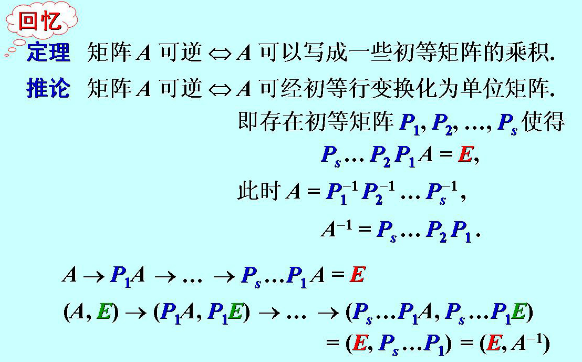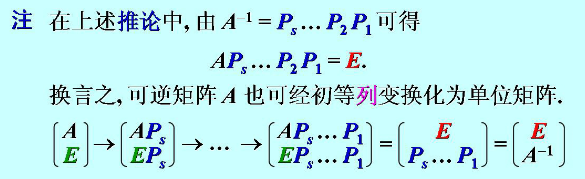2. 逆矩阵的计算示例 （行变换）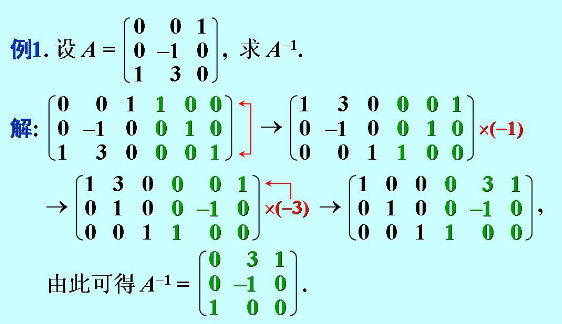3. 逆矩阵的计算示例 （列变换）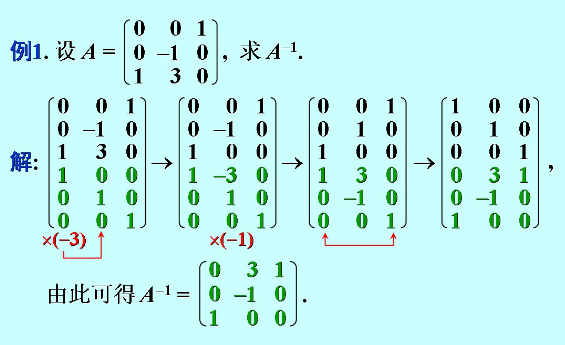4. 利用初等变换求逆矩阵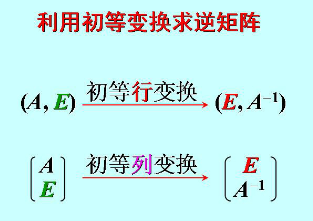展开全文• 逆矩阵初等变换法
大致过程：  - |A|!=0  - 左下角变换0（若对角不为1元素下方元素为1交换两行.）  - 对角元素变1  - 右上角变换0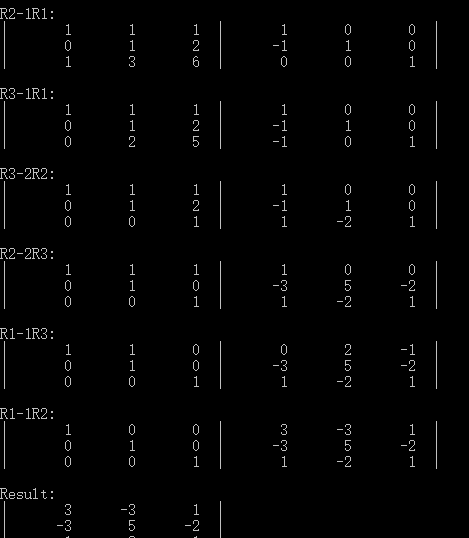源码：
#include<iostream>
#include<iomanip>
using namespace std;
//By Vove.
//左下角变换0 若对角不为1元素下方元素为1交换两行
//对角变1
//右上角变换0
void LD_triangle(float temp,int n);
void RU_triangle(float temp,int n);
void Aii_Cto_1(float temp,int n);
void Display(float A,int m,int n);
int Find(float temp,int i,int n);
void Swap(float temp,int i,int j,int n);

class Matrix{
public:
Matrix(){
n=9;
for(int i=0;i<6;i++)
for(int j=0;j<12;j++)
A[i][j]=0;
Det_A=0;
}
void Conversion();
void Input();
bool Cal_DetA();
friend void Save_To_Result(float temp,Matrix &D);
void Cheak_IsSolvable();
void Out_Result();
private:
int n;                  //阶
float A;         //矩阵
float result;     //逆矩阵结果
float Det_A;            //|A|值 ：是否存在逆矩阵
};

int main(){
cout<<"\n\t\t-------求逆矩阵（初等变换法）-------"<<endl<<endl;
while(1){
Matrix D;
D.Input();
D.Cheak_IsSolvable();   //可解
D.Conversion();         //变换
D.Out_Result();         //输出
}
return 1;
}

void Matrix::Input(){
cout<<"\n---行列数：";
cin>>n;
cout<<endl<<"输入行列式："<<endl;
for(int i=0;i<n;i++)
for(int j=0;j<n;j++)
cin>>A[i][j];
int j=0;
for(i=n;i<2*n;i++){
A[j][i]=1;
j++;
}
}
void Matrix::Cheak_IsSolvable(){
while(1){
if(Cal_DetA()){
cout<<"\n|A|="<<Det_A<<endl<<endl;
cout<<"(A|E)="<<endl;
Display(A,n,2*n);
break;
}
else{
cout<<"此矩阵的行列式为0，无解"<<endl;
Input();
}
}
}
void Matrix::Conversion(){
float temp;
for(int i=0;i<n;i++)//替身
for(int j=0;j<2*n;j++)
temp[i][j]=A[i][j];
LD_triangle(temp,n);//左下角变换0
Aii_Cto_1(temp,n);   //对角变1
RU_triangle(temp,n);//右上角变换
Save_To_Result(temp,*this);
}
void LD_triangle(float temp,int n){
for(int i=0;i<n-1;i++){
if(temp[i][i]){//对角线元素不为0
if(temp[i][i]!=1.0)
if(int j=Find(temp,i,n)) Swap(temp,i,j,n);
for(int m=i+1;m<n;m++){
if(temp[m][i]){
float t=-(temp[m][i])/temp[i][i];
for(int p=0;p<2*n;p++){//R(m)+tRi
temp[m][p]=temp[m][p]+t*temp[i][p];
}
if(t>0)
cout<<"R"<<m+1<<"+"<<t<<"R"<<i+1<<":"<<endl;
else
cout<<"R"<<m+1<<t<<"R"<<i+1<<":"<<endl;
Display(temp,n,2*n);
}
else continue;
}
}

else {//若对角线元素为0
int m;          for(m=i+1;m<n;m++){//
if(temp[m][i]){//使对角线元素非0
for(int p=0;p<n;p++)//Ri+Rm
temp[i][p]=temp[m][p]+temp[i][p];
break;
}
else continue;
}
if(m==n){   //可逆矩阵 不会出现此情况（对角元素为零，且此元素以下全为零）
//此情况 矩阵 行列式值为0
}
cout<<"R"<<i+1<<"+"<<"R"<<m+1<<endl;
Display(temp,n,2*n);
i--;
}
}
}
int Find(float temp,int i,int n){
for(int m=i+1;m<n;m++)
if(temp[m][i]==1.0)
return m;
return 0;
}
void Swap(float temp,int i,int j,int n){
cout<<"R"<<i+1<<"<-->R"<<j+1<<endl;
for(int m=0;m<2*n;m++){
temp[i][m]+=temp[j][m];
temp[j][m]=temp[i][m]-temp[j][m];
temp[i][m]-=temp[j][m];
}
Display(temp,n,2*n);
}
void Aii_Cto_1(float temp,int n){
for(int i=0;i<n;i++){
if(temp[i][i]!=1.0){
float t=1.0/temp[i][i];
cout<<"R"<<i+1<<"/"<<temp[i][i]<<endl;
for(int p=0;p<2*n;p++){//R(m)+tRi
temp[i][p]*=t;
}
Display(temp,n,2*n);
}
}
}
void RU_triangle(float temp,int n){
for(int i=n-1;i>0;i--){
for(int m=i-1;m>=0;m--){
if(temp[m][i]){
float t=-(temp[m][i])/(temp[i][i]);
for(int p=i;p<2*n;p++){ //R(m)+tempRi
temp[m][p]=temp[m][p]+t*temp[i][p];
}
if(t>0)
cout<<"R"<<m+1<<"+"<<t<<"R"<<i+1<<":"<<endl;
else
cout<<"R"<<m+1<<t<<"R"<<i+1<<":"<<endl;
Display(temp,n,2*n);
}
else continue;
}
}
}
void Display(float A,int m,int n){
for(int i=0;i<m;i++){
cout<<"|";
for(int j=0;j<n;j++){
cout<<setw(8)<<A[i][j];
if((j+1)%m==0)
cout<<"  |";
}
cout<<endl;
}
cout<<endl;
}
void Save_To_Result(float temp,Matrix &D){
for(int i=0;i<D.n;i++)
for(int j=D.n;j<2*D.n;j++)
D.result[i][j-D.n]=temp[i][j];
}
bool Matrix::Cal_DetA(){
float temp;
for(int i=0;i<n;i++)
for(int j=0;j<n;j++)
temp[i][j]=A[i][j];
for(i=0;i<n-1;i++){
if(temp[i][i]){//对角线元素不为0
for(int m=i+1;m<n;m++){
if(temp[m][i]){
float tem=-(temp[m][i])/temp[i][i];
for(int p=0;p<n;p++)//R(m)+tempRi
temp[m][p]=temp[m][p]+tem*temp[i][p];
}
else continue;
}
}
else {//若对角线元素为0
int m;
for(m=i+1;m<n;m++){//
if(temp[m][i]){//使对角线元素非0
for(int p=0;p<n;p++)//Ri+Rm
temp[i][p]=temp[m][p]+temp[i][p];
break;
}
else continue;
}
if(m==n){
Det_A=0;
return false;
}
i--;
}
}
Det_A=temp;//求对角积
for(i=1;i<n;i++)
Det_A=Det_A*temp[i][i];

if(Det_A) return true;
else return false;
}
void Matrix::Out_Result(){
cout<<"Result:"<<endl;
for(int i=0;i<n;i++){
cout<<"|";
for(int j=0;j<n;j++){
cout<<setw(8)<<result[i][j];
}
cout<<"  |"<<endl;
}
cout<<endl;
}

展开全文c语言 C++
• P4783 【模板】矩阵求逆 题目描述 一个N×NN×NN×N的矩阵逆矩阵。答案对109+710^9+7109+7取模。 1.逆矩阵的定义 假设 AAA 是一个方阵，如果存在一个矩阵 A−1A^{-1}A−1，使得 A−1A=IA^{-1}A=IA−1A=I 并且 AA...
luogu P4783 【模板】矩阵求逆
题目描述
求一个

N

×

N

N×N

的矩阵的逆矩阵。答案对

1

0

9

+

7

10^9+7

取模。
1.逆矩阵的定义
假设

A

A

是一个方阵，如果存在一个矩阵

A

−

1

A^{-1}

，使得

A

−

1

A

=

I

A^{-1}A=I

并且

A

A

−

1

=

I

AA^{-1}=I

那么，矩阵 A 就是可逆的，

A

−

1

A^{-1}

称为 A 的逆矩阵
2.逆矩阵求法 —— 初等变换法（高斯-约旦消元）
0.高斯-约旦消元
详见P3389 【模板】高斯消元法题解部分
高斯约旦消元与高斯消元区别：
高斯消元 -> 消成上三角矩阵

高斯-约旦消元 -> 消成对角矩阵

约旦消元法的精度更好,代码更简单,没有回带的过程
void Gauss_jordan(){
/***** 行的交换&加减消元 *****/
for(re int i=1,r;i<=n;++i){	//正在处理第i行
r=i;
for(re int j=i+1;j<=n;++j)
if(fabs(a[j][i])>fabs(a[r][i])) r=j;
if(fabs(a[r][i])<eps){
puts("No Solution");return;
}
if(i!=r) swap(a[i],a[r]);

for(re int k=1;k<=n;++k){
//每一行都处理
if(k==i) continue;
double p=a[k][i]/a[i][i];
for(re int j=i;j<=n+1;++j) a[k][j]-=p*a[i][j];
}
}

//上述操作后会剩下对角矩阵,答案要除以系数
for(re int i=1;i<=n;++i) printf("%.2lf\n",a[i][n+1]/a[i][i]);
}


1.矩阵求逆
思路
求

A

A

的逆矩阵，把

A

A

和单位矩阵

I

I

放在一个矩阵里对

A

A

进行加减消元使

A

A

化成单位矩阵此时原来单位矩阵转化成逆矩阵
原理

A

−

1

∗

[

A

I

]

=

[

I

A

−

1

]

A^{-1} * [AI] = [I A^{-1}]

举个栗子 求

[

2

−

1

0

−

1

2

−

1

0

−

1

2

]

\left[ \begin{matrix} 2 &amp; -1 &amp; 0 \\ -1 &amp; 2 &amp; -1 \\ 0 &amp; -1 &amp; 2 \end{matrix} \right]

首先

[

2

−

1

0

1

0

0

−

1

2

−

1

0

1

0

0

−

1

2

0

0

1

]

\begin{bmatrix} 2 &amp; -1 &amp; 0 &amp; 1 &amp; 0 &amp; 0 \\ -1 &amp; 2 &amp; -1 &amp; 0 &amp; 1 &amp; 0 \\ 0 &amp; -1 &amp; 2 &amp; 0 &amp; 0 &amp; 1 \end{bmatrix}

对左边进行消元可得

[

2

−

1

0

1

0

0

0

3

2

−

1

1

2

1

0

0

0

4

3

1

3

2

3

1

]

\left[ \begin{matrix} 2 &amp; -1 &amp; 0 &amp; 1 &amp; 0 &amp; 0 \\ 0 &amp; \frac{3}{2} &amp; -1 &amp; \frac{1}{2} &amp; 1 &amp; 0 \\ 0 &amp; 0 &amp; \frac{4}{3} &amp; \frac{1}{3} &amp; \frac{2}{3} &amp; 1 \end{matrix} \right]

此时已消成上三角矩阵，高斯消元开始回代，但约旦会消成对角矩阵

[

2

0

0

3

2

1

1

2

0

3

2

0

3

4

3

2

3

4

0

0

4

3

1

3

2

3

1

]

\left[ \begin{matrix} 2 &amp; 0 &amp; 0 &amp; \frac{3}{2} &amp; 1 &amp; \frac{1}{2} \\ 0 &amp; \frac{3}{2} &amp; 0 &amp; \frac{3}{4} &amp; \frac{3}{2} &amp; \frac{3}{4} \\ 0 &amp; 0 &amp; \frac{4}{3} &amp; \frac{1}{3} &amp; \frac{2}{3} &amp; 1 \end{matrix} \right]

最后每行除以系数

[

1

0

0

3

4

1

2

1

4

0

1

0

1

2

1

1

2

0

0

1

1

4

1

2

3

4

]

\left[ \begin{matrix} 1 &amp; 0 &amp; 0 &amp; \frac{3}{4} &amp; \frac{1}{2} &amp; \frac{1}{4} \\ 0 &amp; 1 &amp; 0 &amp; \frac{1}{2} &amp; 1 &amp; \frac{1}{2} \\ 0 &amp; 0 &amp; 1 &amp; \frac{1}{4} &amp; \frac{1}{2} &amp; \frac{3}{4} \end{matrix} \right]

此时右半边即为所求
2.细节
开long long（不要冒风险，乘法很容易溢出）模意义下除以一个数等于乘上逆元，可用快速幂求逆元（费马小定理）

C

o

d

e

Code

#include<iostream>
#include<cstdio>
#include<cmath>
#define re register
#define il inline
#define ll long long
using namespace std;

ll s=0,f=0;char c=getchar();
while(c<'0'||c>'9') f=(c=='-'),c=getchar();
while(c>='0'&&c<='9') s=(s<<3)+(s<<1)+(c^'0'),c=getchar();
return f?-s:s;
}

const int N=405,mod=1e9+7;
int n;
ll a[N][N<<1];
il ll qpow(ll x,ll k){
ll ans=1;
while(k){
if(k&1) ans=ans*x%mod;
x=x*x%mod;
k>>=1;
}
return ans%mod;
}

il void Gauss_j(){
for(re int i=1,r;i<=n;++i){
r=i;
for(re int j=i+1;j<=n;++j)
if(a[j][i]>a[r][i]) r=j;
if(r!=i) swap(a[i],a[r]);
if(!a[i][i]){puts("No Solution");return;}

int kk=qpow(a[i][i],mod-2);	//求逆元
for(re int k=1;k<=n;++k){
if(k==i) continue;
int p=a[k][i]*kk%mod;
for(re int j=i;j<=(n<<1);++j)
a[k][j]=((a[k][j]-p*a[i][j])%mod+mod)%mod;
}

for(re int j=1;j<=(n<<1);++j) a[i][j]=(a[i][j]*kk%mod);
//更新当前行 如果放在最后要再求一次逆元,不如直接放在这里
}

for(re int i=1;i<=n;++i){
for(re int j=n+1;j<(n<<1);++j) printf("%lld ",a[i][j]);
printf("%lld\n",a[i][n<<1]);
}
}
int main(){
for(re int i=1;i<=n;++i)
for(re int j=1;j<=n;++j)

Gauss_j();
return 0;
}


网上浏览一圈头都要炸掉，线性代数太可怕了，定义好多 最后只看懂了这种方法
有什么问题欢迎评论区指出 ：）
参考文章
线性代数之——矩阵乘法和逆矩阵
逆矩阵的几种求法与解析(很全很经典)
展开全文• 高等代数的理论知识(A | E) 经过初等变换（E | A-1）（A-1 代表A的）matrix_inv &lt;- function(A) { A_zhi &lt;- Matrix::rankMatrix(A) n_row &lt;- dim(A) n_col &lt;- dim(A) ...
高等代数的理论知识

(A | E) 经过初等行变换（E | A-1）（A-1 代表A的逆）
matrix_inv <- function(A)
{
A_zhi <- Matrix::rankMatrix(A)
n_row <- dim(A)
n_col <- dim(A)
if((n_col==n_row)&(n_row == A_zhi))
{
B <- diag(1,n_col)
col_index <- 1
for(row_index in 1:(n_row - 1))
{
temp_col <- A[col_index:n_row,col_index]

non_zreo_row <- which(temp_col!=0)
#一定找出当前行第一个元素不为零
temp_row <- A[col_index,]
A[col_index,] <-
A[col_index + non_zreo_row - 1,]
A[col_index + non_zreo_row - 1,] <- temp_row
temp_row1 <- B[col_index,]
B[col_index,] <-
B[col_index + non_zreo_row - 1,]
B[col_index + non_zreo_row - 1,] <- temp_row1
#其余的元素化零
for(j in (col_index + 1):n_row)
{
temp_number <-
A[j,col_index]/A[col_index,col_index]
A[j,] <- A[j,] - A[col_index,]*  temp_number
B[j,] <- B[j,] - B[col_index,]* temp_number
}
col_index <- col_index + 1
}
#此是的A时候一个上三角,接下来化为对角矩阵
col_index <- n_col
for(row_index in n_row:2)
{
for(j in (col_index - 1):1)
{
temp_number <-
A[j,col_index]/A[col_index,col_index]
A[j, ] <- A[j, ] - A[col_index,] *
temp_number
B[j,] <- B[j,] - B[col_index,] *
temp_number
}
col_index <- col_index - 1
}
#此是的A时候一个对角矩阵,接下来化为对角矩阵
#标准化
for(i in 1:n_col)
{
B[i,] <- B[i,]/A[i,i]
}
return(MASS::fractions(B))
}
else
{
print("输入的矩阵无法求逆")
}
}

matrix_inv(A)

[,1] [,2] [,3]
[1,]  1    0   -1
[2,] -1    1    1
[3,]  0    0    1

展开全文• 逆矩阵是数学知识的一种，很多学习数学的同学们应该很了解吧。逆矩阵计算器是一款可以对矩阵进行计算的免费程序,本程序引入了分数算法,可以对分数元素...逆矩阵的另外一种常用的求法：(A|E)经过初等变换得到(...
• 如何用MATLAB求逆矩阵如果英文好呢，自己看目录不好还是先看中文的教材，对matlab的框架和功能有了一定的了解后，自己也就看的懂帮助里面的内容了，以后不懂再自己查帮助求逆矩阵一般有2种方法：1、伴随矩阵法。...
• 第一节 矩阵初等变换 一. 数学概念 等价关系具有的性质： (i)　反身性　A~A; (ii) 对称性　若A~B,则B~A; (iii) 传递性　若A~B, B~C,则A~C; 二. 重点，难点分析 本节的重点是用矩阵初等变换将...线性代数 线性方程组
• 文章目录任务详解：1....主要介绍了矩阵初等变换逆矩阵的另外一种求法矩阵的秩，线性方程组的解等知识点。 掌握目标： 1、了解由高斯消元法引入矩阵初等变换 2、掌握矩阵的三种初等变...
• 如下： 转载于:https://www.cnblogs.com/geeksongs/p/11243320.html
• 一种可行的方法是将其与一个对应维度的单位矩阵进行列的拼接,然后对所拼接的矩阵只进行初等的行变换并且当左侧的矩阵变换成为nnn维的单位矩阵时,右侧的矩阵则为待逆矩阵,见式(1-1-1),其中矩阵AAA是一个nnn阶的...算法 线性代数 c语言
• ## 伴随矩阵求逆矩阵

万次阅读 多人点赞 2018-11-15 13:58:08
在之前的文章《线性代数之矩阵》中已经介绍了一些关于矩阵的基本概念，本篇文章主要就求解逆矩阵进行进一步总结。 余子式(Minor) 我们先看例子来直观的理解什么是余子式(Minor，后边将都用英文Minor，中文的翻译较...
• C语言编程中，进行矩阵求逆的计算，运用初等变换原理，为什么我最后的结果中，后3列的数字一直不变呢？0如题：最后的结果一直都是1 0 0 1 0 00 1 0 0 1 00 0 1 0 0 1就是说AE=EB中，后三列的数字一直都是E，即1 0 ...
• 输入矩阵要求为方阵（n×n） 原理：将一个矩阵经过初等变换化为上三角矩阵C++ 算法
• 矩阵的3种运算我们称之为“行初等变换”： 交换任意2行 某一行的元素全部乘以一个非0数 某一行的元素加上另一行对应元素的N倍，N不为0 以矩阵实施行初等变换等同于在矩阵左边乘以一个矩阵。 当要求矩阵A的...
• 矩阵逆求法强行解线性方程法初等变换法伴随矩阵求法很多，下面是几种比较常见的。 强行解线性方程法 把逆矩阵的定义写出来 [a11a12⋯a1na21a22⋯a2n⋯⋯⋯⋯an1an2⋯ann][x11x12⋯x1nx21x22⋯x2n⋯⋯⋯⋯xn...
• 矩阵求逆的思路与方法 逆矩阵的定义 若一个n*n的方阵A可逆，则存在一个n*n的方阵B， 使得。则称B是A的一个逆矩阵。A的逆矩阵记作A-1。 （1）验证两个矩阵互为逆矩阵 矩阵 按照矩阵的乘法满足： 。 ...
• 选主元的高斯-约当（Gauss-Jordan）消元在很多地方都会用到，例如一个矩阵逆矩阵、解线性方程组（插一句：LM算法求解的一个步骤），等等。它的速度不是最快的，但是它非常稳定（来自网上的定义：一个计算方法...
• 2019独角兽企业重金招聘Python工程师标准>>> ...下面的方法求逆矩阵，还蛮不错的。缺点：n行n列时就无法使用了。 转载于:https://my.oschina.net/Bettyty/blog/782265
• 第三节　线性方程组的解 一. 数学概念 根据矩阵的乘法，可以将线性方程组写...原理、公式和法则 定理3.1 n元齐次线性方程组 有非零解的充分必要条件的系数矩阵A的秩 R(A)n。 定理3.2 n元非齐次线性方程组 有线性代数 线性方程组的解
• 打洞原理→分块乘法的初等变换及应用举例 将分块乘法与初等变换结合是矩阵运算中极重要的手段. 现将某个单位矩阵如下进行分块： (EmOOEn)\left(\begin{array}{cc} {E}_{m} & {O} \\ {O} & {E}_{n} \end{...
• 矩阵打洞是高等代数的基本功。 利用分块矩阵的乘法将矩阵的某个块变为零 (ABCD)=(I0CA−1I)(AB0D−CA−1B)\left( \begin{array} { l l } { A } & { B } \\ { C } & { D} \end{array}\right)= \left( \begin{...
• 我们已经了解了对于方程组来说，如何根据他的系数矩阵的变化，来实现高斯-诺尔当消元算法，并可以快速的...以及逆矩阵求法，看看它有什么用把！ 1 逆矩阵 对于任何一个矩阵A来说，若存在一个矩阵B，使得：A∗B...线性代数
• ## 线性代数笔记8——求解逆矩阵

万次阅读 多人点赞 2018-08-24 18:10:32
或许用行列式求逆矩阵的做法有些公式化，实际上可以将求逆矩阵看成解方程组：   　由此可以通过解方程组的方式逆矩阵。 　如果一个方阵与另一个非零矩阵的乘积是零矩阵，那么该矩阵是奇异矩阵，也是就是...
• （1）逆矩阵的唯一性。 若矩阵A是可逆的，则A的逆矩阵是唯一的，并记作A的逆矩阵为A-1...（3）任何一个满秩矩阵都能通过有限次初等变换化为单位矩阵。 推论 满秩矩阵A的逆矩阵A可以表示成有限个初等矩阵的乘积。 ...
• ## 矩阵论（一）：广义逆矩阵（上）

万次阅读 多人点赞 2018-09-11 20:21:22
逆矩阵与右逆矩阵 {1} Moore-Penrose广义 {1,4}与极小范数解 {1,3}与最小二乘解 左逆矩阵与右逆矩阵 我们知道，只有方阵才有逆矩阵，且可逆方阵对方阵是有限制条件的，只有行列式不为零的...机器学习 线性回归
• 原理：应用列主元消去运算矩阵A的逆矩阵，利用初等矩阵变换A转化单位矩阵时，同样的行变化可将单位矩阵转化为A的逆矩阵。 步骤： 编制下三角部分消元和上三角部分消元的代码 a. 从对角线元素往下比较取得这...
• 逆矩阵 编辑讨论 本词条由“科普中国”科学百科词条编写与应用工作...设A是数域上的一个n阶矩阵，若在相同数域上存在另一个n阶矩阵B，使得：AB=BA=E，则我们称B是A的逆矩阵，而A则被称为可逆矩阵。注：E为单位...
• 其次我们要了解矩阵的三种初等变换： 1.交换某两行。 2.将某一行的所有元素乘上k(k≠0)。 3.将某一行的所有元素乘上k加到另一行去。 根据这三种初等变换便可以进行高斯消元。 现将矩阵消成上三角矩阵，再将上三角......